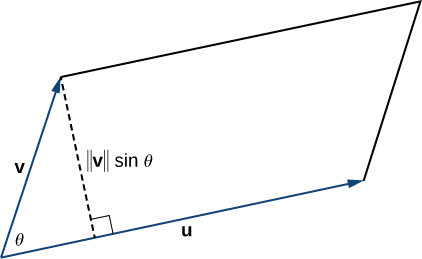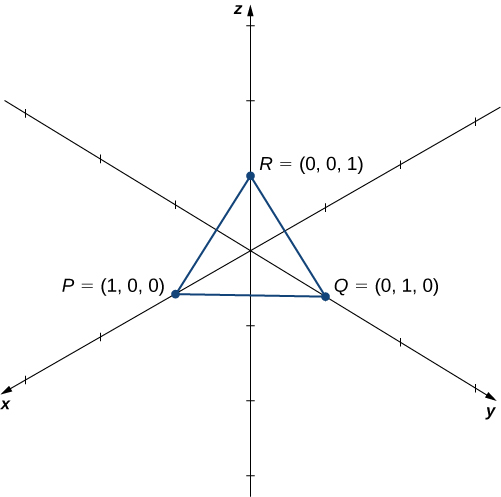# 2.4 The cross product  (Page 4/16)

 Page 4 / 16

## Using expansion along the first row to compute a $3\phantom{\rule{0.2em}{0ex}}×\phantom{\rule{0.2em}{0ex}}3$ Determinant

Evaluate the determinant $|\begin{array}{ccc}\hfill 2& \hfill 5& \hfill -1\\ \hfill -1& \hfill 1& \hfill 3\\ \hfill -2& \hfill 3& \hfill 4\end{array}|.$

We have

$\begin{array}{cc}\hfill |\begin{array}{ccc}\hfill 2& \hfill 5& \hfill -1\\ \hfill -1& \hfill 1& \hfill 3\\ \hfill -2& \hfill 3& \hfill 4\end{array}|& =2|\begin{array}{cc}1\hfill & 3\hfill \\ 3\hfill & 4\hfill \end{array}|-5|\begin{array}{cc}-1\hfill & 3\hfill \\ -2\hfill & 4\hfill \end{array}|-1|\begin{array}{cc}-1\hfill & 1\hfill \\ -2\hfill & 3\hfill \end{array}|\hfill \\ & =2\left(4-9\right)-5\left(-4+6\right)-1\left(-3+2\right)\hfill \\ & =2\left(-5\right)-5\left(2\right)-1\left(-1\right)=-10-10+1\hfill \\ & =-19.\hfill \end{array}$

Evaluate the determinant $|\begin{array}{ccc}\hfill 1& \hfill -2& \hfill -1\\ \hfill 3& \hfill 2& \hfill -3\\ \hfill 1& \hfill 5& \hfill 4\end{array}|.$

$40$

Technically, determinants are defined only in terms of arrays of real numbers. However, the determinant notation provides a useful mnemonic device for the cross product formula.

## Rule: cross product calculated by a determinant

Let $\text{u}=⟨{u}_{1},{u}_{2},{u}_{3}⟩$ and $\text{v}=⟨{v}_{1},{v}_{2},{v}_{3}⟩$ be vectors. Then the cross product $\text{u}\phantom{\rule{0.2em}{0ex}}×\phantom{\rule{0.2em}{0ex}}\text{v}$ is given by

$\text{u}\phantom{\rule{0.2em}{0ex}}×\phantom{\rule{0.2em}{0ex}}\text{v}=|\begin{array}{ccc}\text{i}\hfill & \text{j}\hfill & \text{k}\hfill \\ {u}_{1}\hfill & {u}_{2}\hfill & {u}_{3}\hfill \\ {v}_{1}\hfill & {v}_{2}\hfill & {v}_{3}\hfill \end{array}|=|\begin{array}{cc}{u}_{2}\hfill & {u}_{3}\hfill \\ {v}_{2}\hfill & {v}_{3}\hfill \end{array}|\text{i}-|\begin{array}{cc}{u}_{1}\hfill & {u}_{3}\hfill \\ {v}_{1}\hfill & {v}_{3}\hfill \end{array}|\text{j}+|\begin{array}{cc}{u}_{1}\hfill & {u}_{2}\hfill \\ {v}_{1}\hfill & {v}_{2}\hfill \end{array}|\text{k}.$

## Using determinant notation to find $\text{p}\phantom{\rule{0.2em}{0ex}}×\phantom{\rule{0.2em}{0ex}}\text{q}$

Let $\text{p}=⟨-1,2,5⟩$ and $\text{q}=⟨4,0,-3⟩.$ Find $\text{p}\phantom{\rule{0.2em}{0ex}}×\phantom{\rule{0.2em}{0ex}}\text{q}.$

We set up our determinant by putting the standard unit vectors across the first row, the components of $\text{u}$ in the second row, and the components of $\text{v}$ in the third row. Then, we have

$\begin{array}{cc}\hfill \text{p}\phantom{\rule{0.2em}{0ex}}×\phantom{\rule{0.2em}{0ex}}\text{q}& =|\begin{array}{ccc}\hfill \text{i}& \hfill \text{j}& \hfill \text{k}\\ \hfill -1& \hfill 2& \hfill 5\\ \hfill 4& \hfill 0& \hfill -3\end{array}|=|\begin{array}{cc}\hfill 2& \hfill 5\\ \hfill 0& \hfill -3\end{array}|\text{i}-|\begin{array}{cc}\hfill -1& \hfill 5\\ \hfill 4& \hfill -3\end{array}|\text{j}+|\begin{array}{cc}\hfill -1& \hfill 2\\ \hfill 4& \hfill 0\end{array}|\text{k}\hfill \\ & =\left(-6-0\right)\text{i}-\left(3-20\right)\text{j}+\left(0-8\right)\text{k}\hfill \\ & =-6\text{i}+17\text{j}-8\text{k}.\hfill \end{array}$

Notice that this answer confirms the calculation of the cross product in [link] .

Use determinant notation to find $\text{a}\phantom{\rule{0.2em}{0ex}}×\phantom{\rule{0.2em}{0ex}}\text{b},$ where $\text{a}=⟨8,2,3⟩$ and $\text{b}=⟨-1,0,4⟩.$

$8\text{i}-35\text{j}+2\text{k}$

## Using the cross product

The cross product is very useful for several types of calculations, including finding a vector orthogonal to two given vectors, computing areas of triangles and parallelograms, and even determining the volume of the three-dimensional geometric shape made of parallelograms known as a parallelepiped . The following examples illustrate these calculations.

## Finding a unit vector orthogonal to two given vectors

Let $\text{a}=⟨5,2,-1⟩$ and $\text{b}=⟨0,-1,4⟩.$ Find a unit vector orthogonal to both $\text{a}$ and $\text{b}.$

The cross product $\text{a}\phantom{\rule{0.2em}{0ex}}×\phantom{\rule{0.2em}{0ex}}\text{b}$ is orthogonal to both vectors $\text{a}$ and $\text{b}.$ We can calculate it with a determinant:

$\begin{array}{cc}\hfill \text{a}\phantom{\rule{0.2em}{0ex}}×\phantom{\rule{0.2em}{0ex}}\text{b}& =|\begin{array}{ccc}\hfill \text{i}& \hfill \text{j}& \hfill \text{k}\\ \hfill 5& \hfill 2& \hfill -1\\ \hfill 0& \hfill -1& \hfill 4\end{array}|=|\begin{array}{cc}\hfill 2& \hfill -1\\ \hfill -1& \hfill 4\end{array}|\text{i}-|\begin{array}{cc}\hfill 5& \hfill -1\\ \hfill 0& \hfill 4\end{array}|\text{j}+|\begin{array}{cc}\hfill 5& \hfill 2\\ \hfill 0& \hfill -1\end{array}|\text{k}\hfill \\ & =\left(8-1\right)\text{i}-\left(20-0\right)\text{j}+\left(-5-0\right)\text{k}\hfill \\ & =7\text{i}-20\text{j}-5\text{k}.\hfill \end{array}$

Normalize this vector to find a unit vector in the same direction:

$‖\text{a}\phantom{\rule{0.2em}{0ex}}×\phantom{\rule{0.2em}{0ex}}\text{b}‖=\sqrt{{\left(7\right)}^{2}+{\left(-20\right)}^{2}+{\left(-5\right)}^{2}}=\sqrt{474}.$

Thus, $⟨\frac{7}{\sqrt{474}},\frac{-20}{\sqrt{474}},\frac{-5}{\sqrt{474}}⟩$ is a unit vector orthogonal to $\text{a}$ and $\text{b}.$

Find a unit vector orthogonal to both $\text{a}$ and $\text{b},$ where $\text{a}=⟨4,0,3⟩$ and $\text{b}=⟨1,1,4⟩.$

$⟨\frac{-3}{\sqrt{194}},\frac{-13}{\sqrt{194}},\frac{4}{\sqrt{194}}⟩$

To use the cross product for calculating areas, we state and prove the following theorem.

## Area of a parallelogram

If we locate vectors $\text{u}$ and $\text{v}$ such that they form adjacent sides of a parallelogram, then the area of the parallelogram is given by $‖\text{u}\phantom{\rule{0.2em}{0ex}}×\phantom{\rule{0.2em}{0ex}}\text{v}‖$ ( [link] ).The parallelogram with adjacent sides u and v has base ‖ u ‖ and height ‖ v ‖ sin θ .

## Proof

We show that the magnitude of the cross product is equal to the base times height of the parallelogram.

$\begin{array}{cc}\hfill \text{Area of a parallelogram}& =\text{base}\phantom{\rule{0.2em}{0ex}}×\phantom{\rule{0.2em}{0ex}}\text{height}\hfill \\ & =‖\text{u}‖\left(‖\text{v}‖\text{sin}\phantom{\rule{0.2em}{0ex}}\theta \right)\hfill \\ & =‖\text{u}\phantom{\rule{0.2em}{0ex}}×\phantom{\rule{0.2em}{0ex}}\text{v}‖\hfill \end{array}$

## Finding the area of a triangle

Let $P=\left(1,0,0\right),Q=\left(0,1,0\right),\phantom{\rule{0.2em}{0ex}}\text{and}\phantom{\rule{0.2em}{0ex}}R=\left(0,0,1\right)$ be the vertices of a triangle ( [link] ). Find its area.Finding the area of a triangle by using the cross product.

We have $\stackrel{\to }{PQ}=⟨0-1,1-0,0-0⟩=⟨-1,1,0⟩$ and $\stackrel{\to }{PR}=⟨0-1,0-0,1-0⟩=⟨-1,0,1⟩.$ The area of the parallelogram with adjacent sides $\stackrel{\to }{PQ}$ and $\stackrel{\to }{PR}$ is given by $‖\stackrel{\to }{PQ}\phantom{\rule{0.2em}{0ex}}×\phantom{\rule{0.2em}{0ex}}\stackrel{\to }{PR}‖\text{:}$

$\begin{array}{ccc}\hfill \stackrel{\to }{PQ}\phantom{\rule{0.2em}{0ex}}×\phantom{\rule{0.2em}{0ex}}\stackrel{\to }{PR}& =\hfill & |\begin{array}{ccc}\hfill \text{i}& \hfill \text{j}& \hfill \text{k}\\ \hfill -1& \hfill 1& \hfill 0\\ \hfill -1& \hfill 0& \hfill 1\end{array}|=\left(1-0\right)\text{i}-\left(-1-0\right)\text{j}+\left(0-\left(-1\right)\right)\text{k}=\text{i}+\text{j}+\text{k}\hfill \\ \hfill ‖\stackrel{\to }{PQ}\phantom{\rule{0.2em}{0ex}}×\phantom{\rule{0.2em}{0ex}}\stackrel{\to }{PR}‖& =\hfill & ‖⟨1,1,1⟩‖=\sqrt{{1}^{2}+{1}^{2}+{1}^{2}}=\sqrt{3}.\hfill \end{array}$

The area of $\text{Δ}PQR$ is half the area of the parallelogram, or $\sqrt{3}\text{/}2.$

Find the area of the parallelogram $PQRS$ with vertices $P\left(1,1,0\right),Q\left(7,1,0\right),R\left(9,4,2\right),$ and $S\left(3,4,2\right).$

$6\sqrt{13}$

where we get a research paper on Nano chemistry....?
nanopartical of organic/inorganic / physical chemistry , pdf / thesis / review
Ali
what are the products of Nano chemistry?
There are lots of products of nano chemistry... Like nano coatings.....carbon fiber.. And lots of others..
learn
Even nanotechnology is pretty much all about chemistry... Its the chemistry on quantum or atomic level
learn
da
no nanotechnology is also a part of physics and maths it requires angle formulas and some pressure regarding concepts
Bhagvanji
hey
Giriraj
Preparation and Applications of Nanomaterial for Drug Delivery
revolt
da
Application of nanotechnology in medicine
what is variations in raman spectra for nanomaterials
I only see partial conversation and what's the question here!
what about nanotechnology for water purification
please someone correct me if I'm wrong but I think one can use nanoparticles, specially silver nanoparticles for water treatment.
Damian
yes that's correct
Professor
I think
Professor
Nasa has use it in the 60's, copper as water purification in the moon travel.
Alexandre
nanocopper obvius
Alexandre
what is the stm
is there industrial application of fullrenes. What is the method to prepare fullrene on large scale.?
Rafiq
industrial application...? mmm I think on the medical side as drug carrier, but you should go deeper on your research, I may be wrong
Damian
How we are making nano material?
what is a peer
What is meant by 'nano scale'?
What is STMs full form?
LITNING
scanning tunneling microscope
Sahil
how nano science is used for hydrophobicity
Santosh
Do u think that Graphene and Fullrene fiber can be used to make Air Plane body structure the lightest and strongest. Rafiq
Rafiq
what is differents between GO and RGO?
Mahi
what is simplest way to understand the applications of nano robots used to detect the cancer affected cell of human body.? How this robot is carried to required site of body cell.? what will be the carrier material and how can be detected that correct delivery of drug is done Rafiq
Rafiq
if virus is killing to make ARTIFICIAL DNA OF GRAPHENE FOR KILLED THE VIRUS .THIS IS OUR ASSUMPTION
Anam
analytical skills graphene is prepared to kill any type viruses .
Anam
Any one who tell me about Preparation and application of Nanomaterial for drug Delivery
Hafiz
what is Nano technology ?
write examples of Nano molecule?
Bob
The nanotechnology is as new science, to scale nanometric
brayan
nanotechnology is the study, desing, synthesis, manipulation and application of materials and functional systems through control of matter at nanoscale
Damian
Is there any normative that regulates the use of silver nanoparticles?
what king of growth are you checking .?
Renato
What fields keep nano created devices from performing or assimulating ? Magnetic fields ? Are do they assimilate ?
why we need to study biomolecules, molecular biology in nanotechnology?
?
Kyle
yes I'm doing my masters in nanotechnology, we are being studying all these domains as well..
why?
what school?
Kyle
biomolecules are e building blocks of every organics and inorganic materials.
Joe
can you provide the details of the parametric equations for the lines that defince doubly-ruled surfeces (huperbolids of one sheet and hyperbolic paraboloid). Can you explain each of the variables in the equations?By OpenStaxBy Ryan LoweBy OpenStaxBy OpenStaxBy Katherina jennife...By Dakota BocanBy Christine ZeelieBy Jessica CollettBy Brenna FikeBy